Catalysis

1.* (1995 F 2)

A. Describe one use of catalysts for improving the environment.

There are many possible answers. Here’s a sampling:

•Catalytic converters, which reduce CO, NOx emissions from cars.

•Oil-eating bacteria to clean up spills. (An enzyme in bacteria catalyzes the decomposition of oil.)

•Born-Haber process (converts NH3 to fertilizer.)

B. Describe a catalytic reaction which degrades the environment.

Chlorine radicals catalyze ozone destruction in the upper atmosphere.

J. What does the following fact have to do with catalysis: cyanide ion (CN- is much more toxic per mole than is carbon monoxide?

CN- binds irreversibly to hemoglobin and myoglobin, thereby destroying their catalytic properties (transporting oxygen through the body). CO also blocks the binding of O2 to hemoglobin and myoglobin by binding to the iron. Although it is a stronger ligand than either CN- or O2, it does not destroy the catalytic properties of the oxygen transport proteins. See the online text about hemoglobin for more detail.

2.* (1995 3 2C) Given the following thermodynamic data, is it feasible to find a catalyst to make H2O2 by bubbling O2 into water according to the following equation?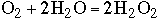O2 + 2H+ + 2e- à H2O2 E0 = +0.70V H202 + 2H+ + 2e- à 2H20 E0 = +1.4V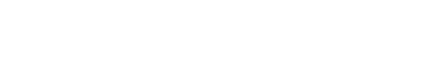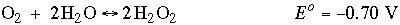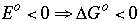Reaction is not thermodynamically favorable / feasible (catalysts can’t change thermodynamics, only kinetics).

3.* (1994 3 4) When a trace of FeSO4 is added to an aqueous solution of H2O2, the solution foams; a gas evolves. When a trace of ZnSO4 is added to another aqueous solution of H2O2, no reaction occurs. Some standard reduction potentials are shown below.

 02 + 4H+ + 4e- à 2H20 E0 = 1.23V 02 + 2H+ + 2e- à H202 E0 = 0.69V H202 + 2H+ + 2e- à 2H20 E0 = 1.78V H02 + H+ + e- à H202 E0 = 1.49V 02 + H+ + e- à H02 E0 = -.105V Zn2+(aq) + 2e- à Zn E0 = -0.76V Fe3+(aq) + 1e- à Fe2+(aq) E0 = 0.77V

1. Write equations showing the reactions that occur when FeSO4 is present.

Reactions that occur when FeSO4 is present: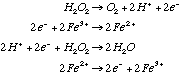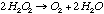The reaction steps must be in this order because the H+'s are required for the second step to occur.

B. Show that the reaction has a negative free energy change.-0.69V +0.77V +1.78V -0.77V +1.09V źG°=-nFE° Because E°>0, źG°<0. The reaction is spontaneous.

C. Explain the role of FeSO4 and the fact that ZnSO4 has no effect. Use balanced equations in your answer. Why is FeSO4 effective even though only a trace amount is used?

FeSO4 acts as a catalyst for this reaction but ZnSO4 does not. Only a trace of FeSO4 is required because, as a catalyst, it is regenerated .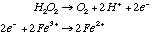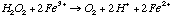-0.69V +0.77V –––– +0.08V The positive E° makes the first step spontaneous.

With Zn: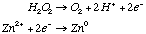-0.69V -0.76V –––– -1.45V The negative E° makes the first step non-spontaneous.

4.* (1990 F 5) Write a paragraph illustrating the principle behind the comparison between a gold brick and a gold-coated brick in the lecture on catalysis.

Although Au is a terrible catalyst, it is a good example. In metal catalysts, all the action happens at the surface. Therefore, below the surface, it really does not matter which atoms are present. Since bricks are cheaper than gold, it is much more cost-efficient to coat a brick with gold than to buy a solid gold brick. (Solid gold bricks are heavy, too!)